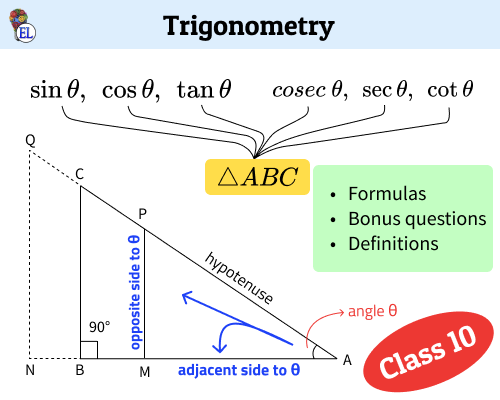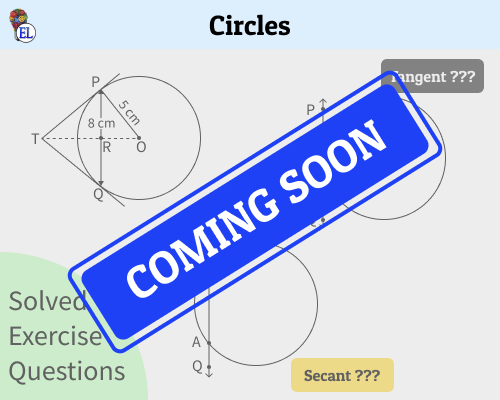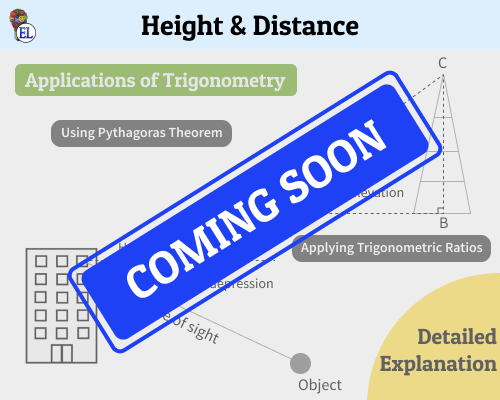# 10th Maths NCERT Important Questions

Trigonometry | Chapter 8 | Maths 10
View questionsCircles | Chapter 10 | Maths 10Height & Distance | Chapter 9 | Maths 10# Trigonometry | Chapter 8 | Maths 10

1. Given a right triangle PQR, where $m \angle Q = 90\degree$, PQ = 12 cm and PR = 13 cm, find the value of $\tan P - \cot R$

2. Given $15 \cot A = 8$, find $\sin A$ and $\sec A$.

3. If 3 cot A = 4, check whether ${1 − \tan^2A \over 1 + \tan^2 A} = \cos^2A - \sin^2A$

4. [Coming Soon] In $\triangle PQR$, right angled at Q, PR + QR = 25cm and PQ = 5cm. Determine the values of $\sin P$, $\cos P$ and $\tan P$.

5. [Coming Soon] Find the value of i) ${\sin 30 \degree + \ \tan 45 \degree - \ \cosec 60 \degree} \over {\sec 30 \degree + \ \cos 60 \degree + \cot 45 \degree}$ ii) Find the value of ${5 \cos ^2 60 \degree + 4 \sec^2 30 \degree - \tan^2 45 \degree \over \sin^2 30 \degree + \cos^2 30 \degree}$